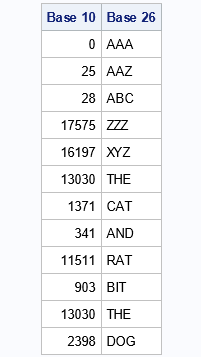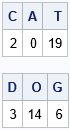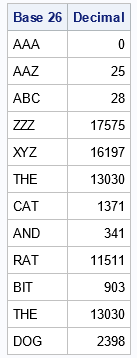I recently showed how to represent positive integers in any base and gave examples of base 2 (binary), base 8 (octal), and base 16 (hexadecimal). One fun application is that you can use base 26 to associate a positive integer to every string of English characters. This article shows how to use base 26 to map integers to strings and vice versa. Here's a sneak peek: the string CAT (base 26) is associated with the integer 2398 (base 10).

### What is base 26?

For any base b, you can express an integer as a sum of powers of b, such as
$\sum\nolimits_{i=0}^p {c_i} b^i$
where the $c_i$ are integers $0 \leq c_i < b$. From the coefficients, you can build a string that represents the number in the given base. Traditionally, we use the symbols 0, 1, ..., 9 to represent the first 10 coefficients and use letters of the English alphabet for higher coefficients. However, in base 26, it makes sense to break with tradition and use English characters for all coefficients. This is done in a natural way by associating the symbols {A, B, C, ..., Z} with the coefficient values {0, 1, 2, ..., 25}. Notice that the symbols for the coefficients in base 26 are zero-based (A=0) and not one-based (A≠1), which is different than you might have seen in other applications.

For example, the number 2398 (base 10) can be written as the sum 3*262 + 14*261 + 6*260. If you use English letters to represent the coefficients, then this number equals DOG (base 26) because 3→D, 14→O, and 6→G. In a similar way, the number 1371 (base 10) can be written as 2*262 + 0*261 + 19*260, which equals CAT (base 26) because 2→C, 0→A, and 19→T.

Recall that for base 10 numbers, we typically do not write the numbers with leading zeros. For example, when considering three-digit numbers in base 10, we do not write the numbers 0-99. But if we use leading zeros, we can write these integers as three-digit numbers: 000, 001, 002, ..., 099. In a similar way, you can represent all three-character strings in base 26 (such as AAA, ABC, and ANT) if you include one or more leading zeros. In base 26, a "leading zero" means that the string starts with A. Unfortunately, if you include leading zeros, you lose a unique representation of the integers because A = AA = AAA, and similarly Z = AZ = AAZ. However, it is a small price to pay. To represent character strings that start with the letter A, you must allow leading zeros.

### A SAS program to represent integers in base 26

It is straightforward to adapt the SAS DATA step program in my previous article to base 26. (See the previous article for an explanation of the algorithm.) In this version, I represent each integer in the range [0, 17575] in base 26 by using a three-digit string. The number 17575 (base 10) is the largest integer that can be represented by using a three-digit string because 17575 (base 10) = 25*262 + 25*261 + 25*260 = ZZZ (base 26).

The following statements put a few integers in the range [0, 17575] into a SAS data set:

/* Example data: Base 10 integers in the [0, 17575] */ data Base10; input x @@; /* x >= 0 */ datalines; 0 25 28 17575 16197 13030 1371 341 11511 903 13030 2398 ;

The following DATA step converts these integers to three-character strings in base 26.

/* For simplicity, only consider three-digit strings. The strings will contain 'leading zero', which means strings like ABC (base 26) = 28 (base 10) Three-digit strings correspond to integers 0 - 17575. */ %let maxCoef = 3; /* number of characters in string that represents the number */ %let base = 26; /* base for the representation */ %let valueList = ('A' 'B' 'C' 'D' 'E' 'F' 'G' 'H' 'I' 'J' 'K' 'L' 'M' 'N' 'O' 'P' 'Q' 'R' 'S' 'T' 'U' 'V' 'W' 'X' 'Y' 'Z');   data Base26; array values[&base] $_temporary_ &valueList; /* characters to use to encode values */ array c[0:%eval(&maxCoef-1)] _temporary_; /* integer coefficients c, c, ... */ length ID$&maxCoef; /* string for base b */ b = &base; /* base for representation */   set Base10; /* x is a positive integer; represent in base b */ /* compute the coefficients that represent x in Base b */ y = x; do k = 0 to &maxCoef-1; c[k] = mod(y, b); /* remainder when r is divided by b */ y = floor(y / b); /* whole part of division */ substr(ID,&maxCoef-k,1) = values[c[k]+1]; /* represent coefficients as string */ end; drop b y k; run;   proc print data=Base26 noobs label; label x="Base 10" ID="Base 26"; var x ID; run;After converting a few arbitrary base 10 numbers to base 26, I show a few special numbers that correspond to three-character English words. The last seven numbers in the data set are integers that, when represented in base 26, correspond to the sentence THE CAT AND RAT BIT THE DOG!

### Convert from base 26 to base 10

In the previous section, I used base 10 numbers that converted to a complete English sentence: THE CAT AND RAT BIT THE DOG. Obviously, I started with the sentence that I wanted to "find," and then figured out which decimal digits would produce the sentence. In other words, I started with the base 26 representation and computed the base 10 (decimal) representation.

For compactness, I will use SAS/IML software to compute the integer value for each base 26 representation. You could use the DATA step, if you prefer. The SAS/IML language supports a little-known feature (the MATTRIB statement) that enables you to index a matrix by using a string instead of an integer. This enables you to put the decimal numbers 0-25 into an array and index them according to the corresponding base 26 characters. This feature is demonstrated in the following example:

proc iml; n = 0:25; /* decimal values */ L = "A":"Z"; /* base 26 symbols */ mattrib n[colname=L]; /* enable indexing n by the letters A-Z */ C = n["C"]; A = n["A"]; T = n["T"]; print C A T; D = n["D"]; O = n["O"]; G = n["G"]; print D O G;The output shows that an expression like n['D'] results in the numerical value of the symbol 'D'. For any base 26 string, you can use the SUBSTR function to extract each character in the string. You can use the MATTRIB trick to find the corresponding base 10 value. You can use the position of each character and the definition of base 26 to find the integer represented by each string:

/* convert base 26 strings to integers */ str = {"AAA" "AAZ" "ABC" "ZZZ" "XYZ" "THE" "CAT" "AND" "RAT" "BIT" "THE" "DOG"}; Decimal = j(nrow(str),1); do j = 1 to nrow(str); s = str[j]; /* get the j_th string */ k = length(s); /* how many characters? */ x = 0; do i = 0 to k-1; /* for each character in the string */ c = substr(s, k-i, 1); /* extract the character at position k-i */ x = x + n[c]*26**i; /* use n[c] as the coefficient in the base 26 representation */ end; Decimal[j] = x; /* save the integer value for the j_th string */ end;   print str[L="Base 26"] Decimal;`The output shows the base 10 representation for a set of three-digit base 26 strings. These are the values that I used in the first part of this article. (NOTE: You can vectorize this program and eliminate the inner loop! I leave that as an exercise.)

### Summary

This article shows a fun fact: You can use base 26 to associate an integer to every string of English characters. In base 26, the string 'CAT' is associated with 2398 (base 10) and the string 'DOG' is associated with the number 1371 (base 10). This article uses three-digit character strings to demonstrate the method, but the algorithms apply to character strings that contain an arbitrary number of characters.

The post Base 26: A mapping from integers to strings appeared first on The DO Loop.